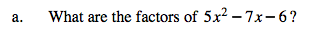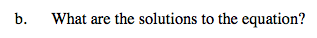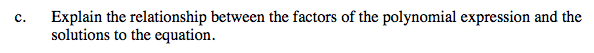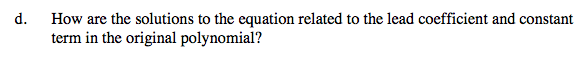### Home > A2C > Chapter Ch9 > Lesson 9.3.1 > Problem9-126

9-126.(5x + 3)(x − 2)Use the factors to find the values.

$\textit{x}=2,\,-\frac{3}{5}$The relationship is that the solutions are the numbers that would make the factors equal to 0.3 and 2 are factors of 6 while 5 is a factor of the lead coefficient.